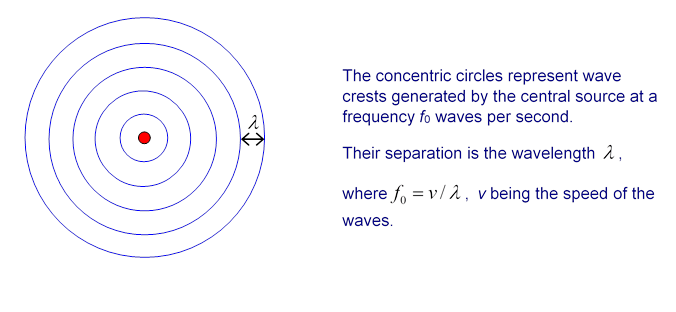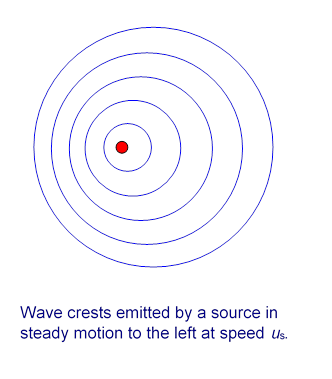# The Doppler Effect

Michael Fowler, University of Virginia

### Introduction

JavaScript Applet here.

The Doppler effect is the perceived change in frequency of sound emitted by a source moving relative to the observer: as a plane flies overhead, the note of the engine becomes noticeably lower, as does the siren noise from a fast-moving emergency vehicle as it passes.  The effect was first noted by Christian Doppler in 1842.  The effect is widely used to measure velocities, usually by reflection of a transmitted wave from the moving object, ultrasound for blood in arteries, radar for speeding cars and thunderstorms.  The velocities of distant galaxies are measured using the Doppler effect (the red shift).

### Sound Waves from a Source at Rest

To set up notation, a source at rest emitting a steady note generates circular wavecrests:The circles are separated by one wavelength $λ$ and they travel outwards at the speed of sound $v.$ If the source has frequency $f 0 ,$ the time interval $τ 0$ between wave crests leaving the source

$τ 0 = 1 f 0 .$

As a fresh wave crest is emitted, the previous crest has traveled a distance $λ,$ so, since it’s moving at speed $v,$

and therefore

$λ f 0 =v.$

### Sound Waves from a Moving Source

The Doppler effect arises because once a moving source emits a circular wave (and provided the source is moving at less than the speed of the wave) the circular wave crest emitted continues its outward expansion centered on where the source was when the crest was emitted, independent of any subsequent motion of the source.

Therefore, if the source is moving at a steady speed, the centers of the emitted circles of waves will be equally spaced along its path, indicating its recent history.  In particular, if the source is moving steadily to the left, the wave crests will form a pattern:Or, to be more realistic (from Wikipedia Commons):It is evident that, as a result of the motion of the source, waves emitted in the forward direction (to the left in the diagram) have a shorter wavelength than they would have if the same source were at rest.  And it’s easy to understand why.

Denoting the steady source velocity by $u s$ , in the time $τ 0 =1/ f 0$  between crests being emitted the source will have moved to the left a distance $u s τ 0 .$  At the same time, the previously emitted crest will itself have moved to the left a distance $λ.$  Therefore, the actual distance between crests emitted to the left will be

$λ ′ =λ− u s τ 0 .$

These waves, having left the source, are of course moving at the speed of sound $v$ relative to the air$—$the motion of the source does not affect the speed of sound in air.  Therefore, as these waves of wavelength $λ ′$ arrive at an observer placed to the left, so the source is moving directly towards him, he will hear a frequency $f ′ =v/ λ ′ .$

### Frequency Detected by Stationary Observer of Moving Source

From the above argument, the observed frequency for a source moving towards the observer at speed $u s$ is:

$f ′ = v λ ′ = v λ− u s τ 0 = v λ ( 1 1− u s τ 0 /λ )= f 0 ( 1 1− u s /v ).$

(Note that for the common case $( u s /v )≪1,$, we can approximate, $f ′ ≅ f 0 ( 1+ u s /v )$.)

By an exactly parallel argument, for a source moving away from an observer at speed $u s ,$ the frequency is lower by the corresponding factor:

### Stationary Source, Moving Observer

Consider now an observer moving at speed $u obs$ directly towards a  stationary frequency $f 0$ source.  So, she’s moving to meet the oncoming wave crests.  Remember, the wave crests are $λ$ apart in the air, and moving at $v.$  Suppose her time between meeting successive crests is $τ ′ .$  During this time, she moves $u obs τ ′ ,$ the wave crest moves $v τ ′$ coming to meet her, and between them they cover the distance $λ$ between crests.It is evident from the diagram that the time interval she will measure between meeting successive crests is

$τ ′ = λ u obs +v$

and therefore the sound frequency she measures is

$f ′ = 1 τ ′ = u obs +v λ = v λ ( 1+ u obs v )= f 0 ( 1+ u obs v ).$

### Source and Observer Both Moving Towards Each Other

For this case, the arguments above can be combined to give:

$f ′ = f 0 ( 1+ u obs /v 1− u s /v ).$

Both motions increase the observed frequency.  If either observer or source is moving in the opposite direction, the observed frequency is found by switching the sign of the corresponding $u.$

### Doppler Effect for Light

The argument above for the Doppler frequency shift is accurate for sound waves and water waves, but fails for light and other electromagnetic waves, since their speed is not relative to an underlying medium, but to the observer.  To derive the Doppler shift in this case requires special relativity.  A derivation can be found in my Modern Physics notes.

The Doppler shift for light depends on the relative velocity $u$ of source and observer (it's relativity, right?):

$f ′ = f 0 1+u/c 1−u/c$

for motion towards each other.

### Other Possible Motions of Source and Observer

We’ve assumed above that the motions of source and observer are all along the same straight line.  But as we hear the change in frequency of a jet engine passing overhead, the note drops smoothly, because we’re off the straight line path of the plane.  The actual note heard as a function of time can be found from fairly simple geometric considerations to be $f ′ = f 0 /( 1− u s cosθ/v ),$ where $θ$ is the angle between the straight line path and a line from the source to the observer.  (Note: if you watch and listen to a jet plane passing overhead, it’s very obvious that the sound you are hearing at any instant is coming from a point the jet left some time ago$—$the jet has traveled a significant distance since it emitted that sound!  So we’re talking here about a line from the observer to the point where the sound was emitted, where a blind man would place the plane. This so-called retardation effect is important here because the jet plane is traveling at a significant fraction of the speed of sound.  It’s irrelevant for police radar units$—$and they do use the formula!)

Notice that if $θ=π/2, f ′ = f 0 .$  This seems very reasonable, but is not the case for light, if the frequency shift is measured accurately enough to find effects of order $( v/c ) 2 .$  To this order, relativistic time dilation of the source gives a frequency shift.  This was found unequivocally in a beautiful series of experiments in the 1930’s (by Ives and Stillwell) who were attempting to establish the opposite:  they were trying to disprove special relativity.